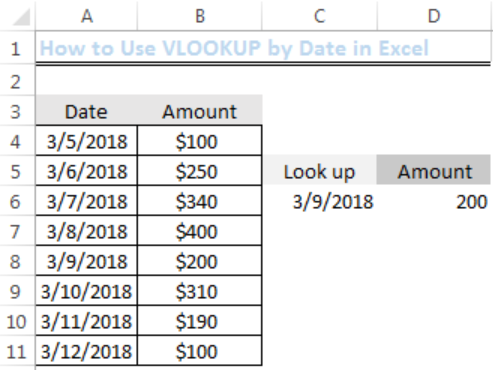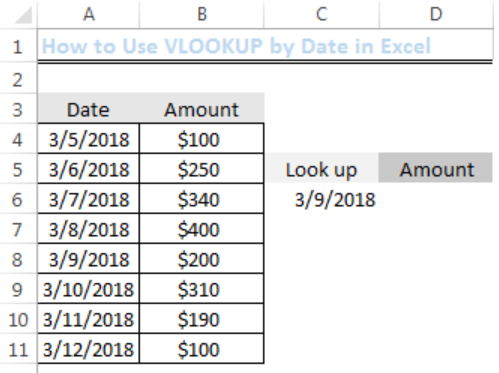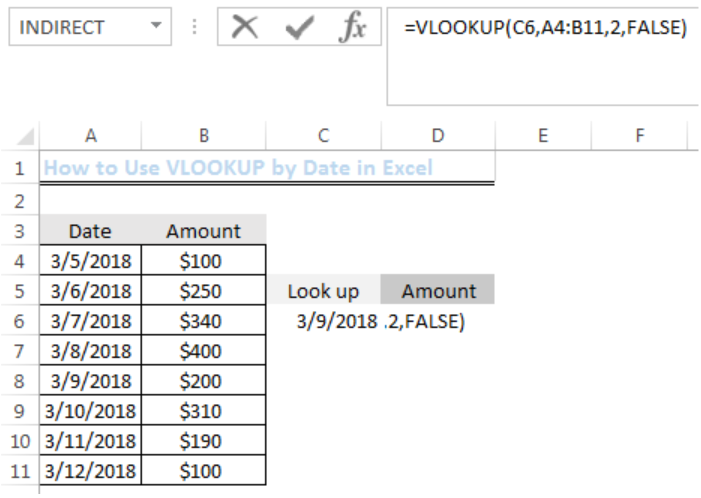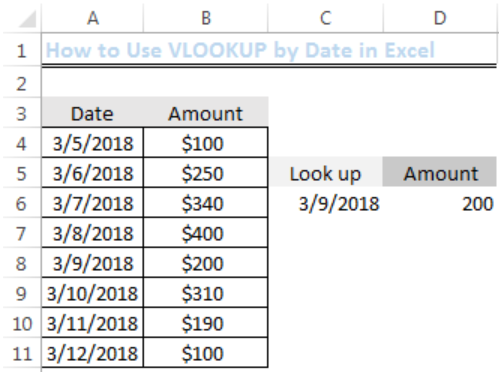Get instant live expert help with Excel or Google Sheets“My Excelchat expert helped me in less than 20 minutes, saving me what would have been 5 hours of work!”

#### Post your problem and you’ll get Expert help in seconds.

Your message must be at least 40 characters
Our professional Expert are available now. Your privacy is guaranteed.

# How to Use VLOOKUP by Date in Excel

We can find values (numbers or text) that are associated with particular dates in excel by using the VLOOKUP function. We can do this by following the easy steps below.Figure 1: The Result of the Lookup Date

## Setting up the Data

We need to have a table to make use of the VLOOKUP function. This table will contain the Dates in Column A and the Amounts (this could be text) in Column B.Figure 2: Setting up the Data

## Syntax

`=VLOOKUP(lookup_value,table_array,col_index_number,{range_lookup})`

## Explanation

• Lookup Value

This is the particular date that we are interested in. For instance, if we are interested in 3/9/2018, our Lookup Value will be A8 because that date is in Cell A8.

• Table Array

This is the range of values from where we will retrieve our value contained in the data. It is noteworthy that we must have at least two columns of data. Our range, in this case, is A4:B11.

• Column Index Number

This is the number of columns from where we will retrieve our data. In this case, we have just two columns, hence, our column index number is 2.

• Range Lookup

This will return values as TRUE or FALSE. TRUE means that we want an approximate match for the selected date and FALSE implies that we want an exact match. We will opt for an exact match.

## Look up Value for 3/9/2018

We will name a Cell that will contain the date and have an empty cell where our formula will return our lookup value.

• Cell C5 will be called lookup
• Cell D5 will be called amount
• Cell C6 will contain the lookup date
• Cell D6 is where we will input our formulaFigure 3: Using the VLOOKUP function

Based on the explanation of the syntax above, our formula for 3/9/2018 is:

`=VLOOKUP(C6,A4:B11,2,FALSE)`

We will input this formula into Cell D6Figure 4: Inserting the VLOOKUP function

We will now press ENTER to get the result.Figure 5: Result of the VLOOKUP

The result has provided the amount for the selected lookup date as shown in figure 5.

When using this function, the dates in the table array and the date in the lookup value must be valid.

## Instant Connection to an Expert through our Excelchat Service

Most of the time, the problem you will need to solve will be more complex than a simple application of a formula or function. If you want to save hours of research and frustration, try our live Excelchat service! Our Excel Experts are available 24/7 to answer any Excel question you may have. We guarantee a connection within 30 seconds and a customized solution within 20 minutes.

Are you still looking for help with the VLOOKUP function? View our comprehensive round-up of VLOOKUP function tutorials here.

### Did this post not answer your question? Get a solution from connecting with the expert.Another blog reader asked this question today on Excelchat:
Solution examplesI filtered data in column A (it is labeled ID #) of a data set. On my next worksheet, column A is also the ID #, but it is a different data set. I want to filter the ID #'s the same for the two sheets, but how do I do that being that I am working with two different sets of data?
Solved by V. H. in 22 minsI'm looking for a formula or format for if there is data in c4 on a sheet... It adds extra information underneath
Solved by X. E. in 20 minshi, my vlookup wont work in some rows but does in others. its temperemental. e.g it will work on rows 10 to 15, then not row 16 and 17, but the will for the rest.
Solved by K. Q. in 21 minsUse the Vlookup Function to complete the "employee" column of table 2. Use "job Id" from table 2 as your lookup_value(s) and table 1 as your reference.
Solved by C. H. in 16 minsIf a cell in another sheet is populated I need a vlookup done. If the cell is not populated I need the cell to return blank.
Solved by T. D. in 60 mins## Subscribe to Excelchat.coAnother blog reader asked this question today on Excelchat: